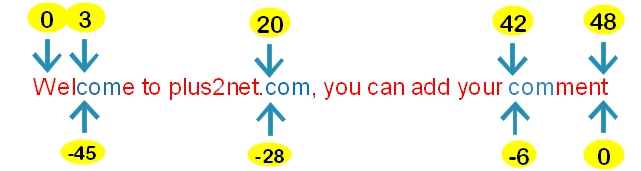# substr_count(): counting presence of sub string inside a main string.

``````\$my_string="PHP is a server side script and learn PHP at www.plus2net.com";
echo substr_count(\$my_string,"PHP");``````
The above code will print 2

Syntax
``substr_count(string \$haystack,string \$needle,int \$offset, int \$length);``
ParameterDESCRIPTION
\$haystackRequired : Input string
\$needleRequired : substring to be searched within Input string
\$offsetOptional : Where to start counting. If negative (in PHP 7 only ) then from end of the input string
\$lengthOptional : The maximum length after the \$offset to search for the substring. If negative ( in PHP 7 only ) then count from end of the haystack ( input string ) only.
Sum of offset and \$length should not be more than the lenght of \$haystack ( Warning as output )
The outpus is integer, based on the number of matches found.

Within a string we can count number of occurrence of another string by using substr_count function. This function takes the main string and the search string as inputs and returns number of time search string is found inside the main string.Use the above string to match the outputs given.
``````\$string="Welcome to plus2net.com, you can add your comment";
echo strlen(\$string); // Output is 49 ( length of the string )
echo substr_count(\$string,'com');       // Output is 3
echo substr_count(\$string,'com',3);     // Output is 3
echo substr_count(\$string,'com',4);     // Output is 2
echo substr_count(\$string,'com',20);     // Output is 2
echo substr_count(\$string,'com',21);     // Output is 1
echo substr_count(\$string,'com',3,3);  // Output is is 1
echo substr_count(\$string,'com',4,3);  // Output is is 0
echo substr_count(\$string,'com',0,10);  // Output is 1

echo substr_count(\$string,'com',4,18);  // Output is 0
echo substr_count(\$string,'com',4,19);  // Output is 1
echo substr_count(\$string,'com',4,18);  // Output is 0
echo substr_count(\$string,'com',20);    // Output is 2
echo substr_count(\$string,'com',20,29); // Output is 2
echo substr_count(\$string,'com',20,30);   // Warning as 20 + 30 is greater than 49``````

## PHP 7 and negative \$offset and \$length

PHP 7 supports negative value for \$offset and \$length ( \$length can be 0 also ) .

Try these codes in PHP 7
``````\$string="Welcome to plus2net.com, you can add your comment";
//echo strlen(\$string); // Output is 49 ( length of the string )
///////// PHP 7 //////////////
echo substr_count(\$string,'com',-49);    // Output is 3
echo substr_count(\$string,'com',-49,-4); // Output is 3
echo substr_count(\$string,'com',-49,-5); // Output is 2
echo substr_count(\$string,'com',-47,-4); // Output is 3
echo substr_count(\$string,'com',-49,-7); // Output is 2
echo substr_count(\$string,'com',-49,-26);// Output is 2
echo substr_count(\$string,'com',-49,-27);// Output is 1
echo substr_count(\$string,'com',-29,-26);// Output is 1
echo substr_count(\$string,'com',-28,-26);// Output is 0``````
Another useful requirement of this functions is calculating how many email address entered by the user in an input field. If you are using one form and sending the form by email where to address is entered by the user. Spammers can use this by placing more than one email address inside the input field. We can count the number of @ used within the input field and display error message. Here is the code

``````if(substr_count(\$y_email,"@") > 1  ){
\$msg .="Use only one email address<BR>";
}``````

Subscribe to our YouTube Channel here

## Subscribe

* indicates required
Subscribe to plus2netplus2net.com

 Paul, copySnips.com 02-09-2009 Thanks for sharing this example. Very useful.
 John 13-10-2014 Very useful thanks!